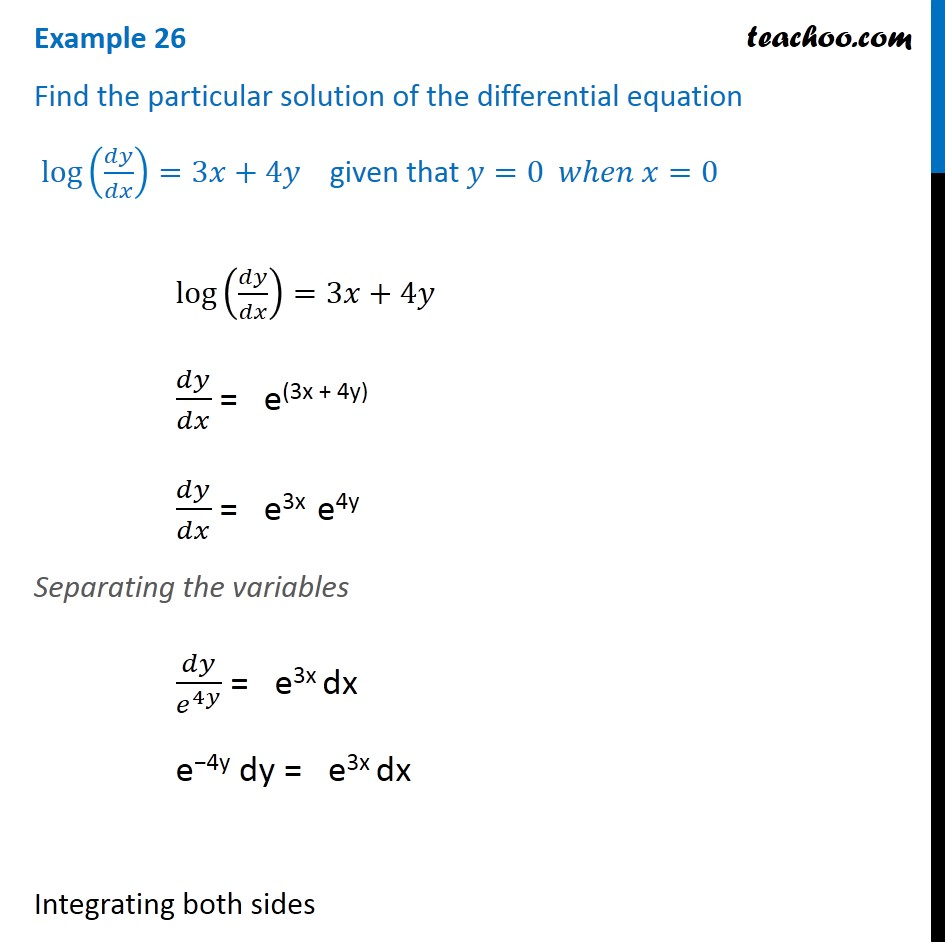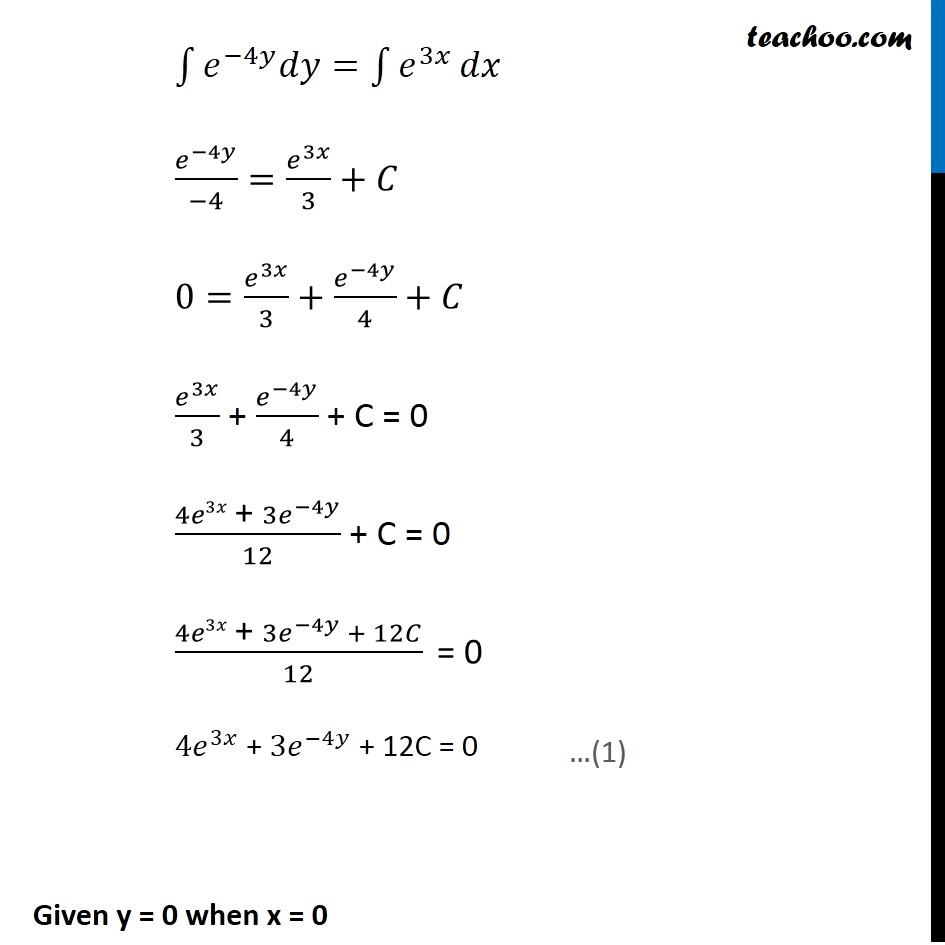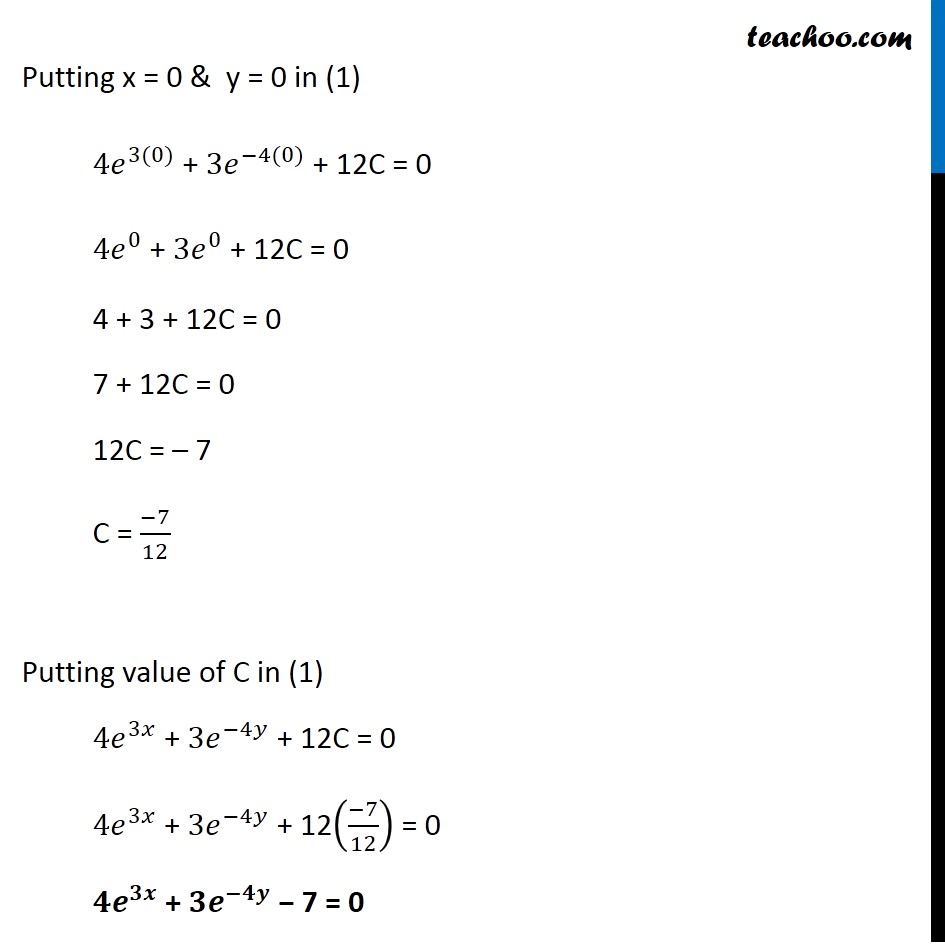Examples

Chapter 9 Class 12 Differential Equations
Serial order wiseLearn in your speed, with individual attention - Teachoo Maths 1-on-1 Class

### Transcript

Example 20 Find the particular solution of the differential equation log(𝑑𝑦/𝑑𝑥)=3𝑥+4𝑦 given that 𝑦=0 𝑤ℎ𝑒𝑛 𝑥=0 log(𝑑𝑦/𝑑𝑥)=3𝑥+4𝑦 𝑑𝑦/𝑑𝑥 = e(3x + 4y) 𝑑𝑦/𝑑𝑥 = e3x e4y Separating the variables 𝑑𝑦/𝑒^4𝑦 = e3x dx e−4y dy = e3x dx Integrating both sides ∫1▒〖𝑒^(−4𝑦) 𝑑𝑦〗=∫1▒𝑒^3𝑥 𝑑𝑥 𝑒^(−4𝑦)/(−4)=𝑒^3𝑥/3+𝐶 0=𝑒^3𝑥/3+𝑒^(−4𝑦)/4+𝐶 𝑒^3𝑥/3 + 𝑒^(−4𝑦)/4 + C = 0 (4𝑒3𝑥" + " 3𝑒^(−4𝑦))/12 + C = 0 (4𝑒3𝑥" + " 3𝑒^(−4𝑦) + 12𝐶)/12 " = 0" 4𝑒^3𝑥 + 3𝑒^(−4𝑦) + 12C = 0 Given y = 0 when x = 0 …(1) Putting x = 0 & y = 0 in (1) 4𝑒^(3(0)) + 3𝑒^(−4(0)) + 12C = 0 4𝑒^0 + 3𝑒^0 + 12C = 0 4 + 3 + 12C = 0 7 + 12C = 0 12C = – 7 C = (−7)/12 Putting value of C in (1) 4𝑒^3𝑥 + 3𝑒^(−4𝑦) + 12C = 0 4𝑒^3𝑥 + 3𝑒^(−4𝑦) + 12((−7)/12) = 0 𝟒𝒆^𝟑𝒙 + 𝟑𝒆^(−𝟒𝒚) − 7 = 0